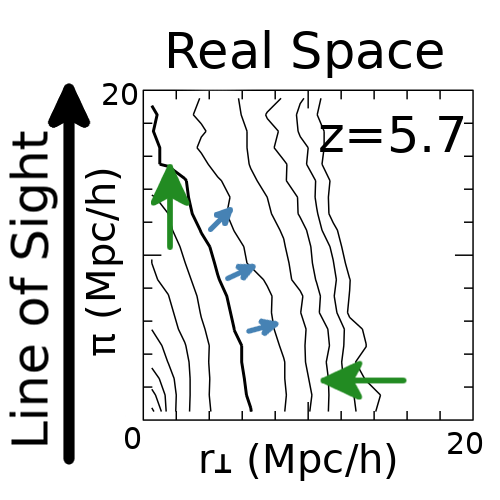# Lya RT Real Space Clustering

### Abstract

Lyman-$\alpha$ emitters (LAEs) are a promising probe of the large-scale structure at high redshift, $z\gtrsim 2$. In particular, the Hobby-Eberly Telescope Dark Energy Experiment aims at observing LAEs at 1.9 $<z<$ 3.5 to measure the Baryon Acoustic Oscillation (BAO) scale and the Redshift-Space Distortion (RSD). However, (Zheng et al. 2011) pointed out that the complicated radiative transfer (RT) of the resonant Lyman-$\alpha$ emission line generates an anisotropic selection bias in the LAE clustering on large scales, $s\gtrsim 10\,{\rm Mpc}$. This effect could potentially induce a systematic error in the BAO and RSD measurements. Also, (Croft et al. 2016) claims an observational evidence of the effect in the Lyman-$\alpha$ intensity map, albeit statistically insignificant. We aim at quantifying the impact of the Lyman-$\alpha$ RT on the large-scale galaxy clustering in detail. For this purpose, we study the correlations between the large-scale environment and the ratio of an apparent Lyman-$\alpha$ luminosity to an intrinsic one, which we call the “observed fraction”, at $2<z<6$. We apply our Lyman-$\alpha$ RT code by post-processing the full Illustris simulations. We simply assume that the intrinsic luminosity of the Lyman-$\alpha$ emission is proportional to the star formation rate of galaxies in Illustris, yielding a sufficiently large sample of LAEs to measure the anisotropic selection bias. We find little correlations between large-scale environment and the observed fraction induced by the RT, and hence a smaller anisotropic selection bias than what was claimed by (Zheng et al. 2011). We argue that the anisotropy was overestimated in the previous work due to the insufficient spatial resolution: it is important to keep the resolution such that it resolves the high density region down to the scale of the interstellar medium, $\sim1$ physical kpc. We also find that the correlation can be further enhanced by assumptions in modeling intrinsic Lyman-$\alpha$ emission.

Type
Publication
The impact of Lyman-α radiative transfer on large-scale clustering in the Illustris simulation
Date(Zheng et al. 2011) For the Lyman-$\alpha$ emission line (Zheng et al. 2011) found a further distortion effect arising from radiative transfer that occurs in real space (and is therefore a selection effect). In numerical simulations this effect was found to show up in the clustering signal by elongation along the line of sight and a decreased bias. This effect can be attributed to correlations of the escape fraction of photons through the IGM with the density and velocity gradient. Given these correlations, the distortions effect are not just limited to small scales, but need to be accounted for in studies aiming to constrain the cosmological model from the large scale modes in Lyman-$\alpha$ galaxy surveys and intensity maps, such as in the HETDEX survey.
While we demonstrated in this paper that there is no radiative transfer distortion effect in our simulations, this does not exclude the possible existence of such effect in reality: The effect strongly depends on the spectrum on leaving the CGM. Dust and clumpiness might strongly effect this spectrum and thus the strength of a possible distortion effect. Future improvements in understanding in the multi-scale modeling (ISM/CGM/IGM) of Lyman-$\alpha$ emitter observations will help settling this question.